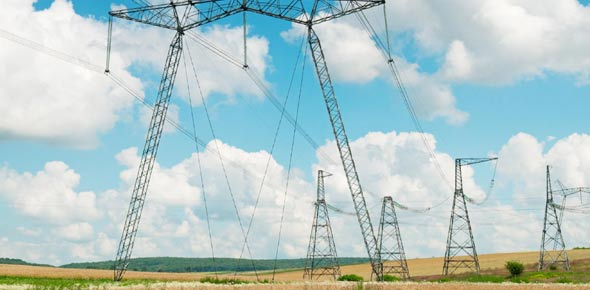# Electrical Quantity Quiz

16 QuestionsSettings.

Related Topics
• 1.
Name two sources of electricity
• A.

Batteries and dynamoes

• B.

Sun and moon

• C.

Candle and bulb

• D.

All the above

• 2.
E.m.f means electromotive force?
• A.

True

• B.

False

• 3.
E.m.f. is the work done by the force acting on charged particles to convert other forms of energy to electrical energy
• A.

True

• B.

False

• 4.
Which below is a device which converts electrical energy into thermal energy
• A.

Electrical heaters

• B.

Electric stoves and electrical kettle

• C.

Hair dryers

• D.

All the above

• 5.
Which device does not change electric energy into light?
• A.

Bulb

• B.

• C.

Tv

• D.

Earphone

• 6.
In an electric motor electrical energy is converted to kinetic energy
• A.

True

• B.

False

• 7.
Potential difference V is defined as the amount of work done W (or energy E converted) to drive a unit charge Q through any two points in a circuit
• A.

True

• B.

False

• 8.
V=W/Q=E/Q, which meaning of the symbols is wrong?
• A.

V = VOLTS

• B.

W = WORK

• C.

Q= CHARGE

• D.

E= EMPTY

• 9.
The unit of electrical power is the joule?
• A.

True

• B.

False

• 10.
One kwh is is one thousand watts burning for one hour or 3600 seconds
• A.

True

• B.

False

• 11.
240 V at 1200 Watts If electricity costs 16 cents per unit, what is the cost of using the iron at maximum power 30 minu tes every day in the month of May (31 days)?
• A.

\$1.00

• B.

\$3.00

• C.

\$2.97

• D.

\$4.00

• 12.
What are 3 ways to conserve electricity
• A.

Turn off unused lights

• B.

Use energy saving bulbs

• C.

Use cold water instead of hot water

• D.

All the above

• 13.
Which of the following bulbs is the most energy efficient?
• A.

Incandescent bulb

• B.

Fluorescent bulb

• C.

Spiral bulbs

• D.

LED bulbs

• 14.
Solar waters heaters save energy by using free sunlight to heat water instead of paying for current
• A.

True

• B.

False

• 15.
Check off all alternative energy sources
• A.

Wind

• B.

Tidal

• C.

Gasolene

• D.

Solar poser

• 16.
How is fossil fuel used to rotate turbine blades to produce electricity?
• A.

Fuel is burnt

• B.

The heat is used to boil water

• C.

The steam pressure is used to spin turbine blades

• D.

The turbine spins the generator and current is produced